Boletín de la Sociedad Geológica Mexicana Volumen 67, núm. 3, 2015, p. 421-432 http://dx.doi.org/10.18268/BSGM2015v67n3a6An interpretation of the oligomerization of amino acids under prebiotic conditions

Fernando G. Mosqueira P. S.1,*, Alicia Negrón-Mendoza2, Sergio Ramos-Bernal2

1 Dirección General de Divulgación de la Ciencia, Universidad Nacional Autónoma de México. Cd. Universitaria, A.p. 70-487, 04510 México, D.F., México.
2 Instituto de Ciencias Nucleares, Universidad Nacional Autónoma de México. Cd. Universitaria, A.p. 70 -543, 04510 México, D.F., México.

* This email address is being protected from spambots. You need JavaScript enabled to view it.

Abstract

In the present work we address to the oligomerization of amino acids under plausible prebiotic conditions and within the framework of a simple stochastic mathematical model. A main premise of our approach is that the reactivity of such monomers is different, as experimental results suggest. Such condition would lead to the synthesis of random but biased polymers and not to purely random polymers. Another manner to phrase such result is to say that synthesized prebiotic oligopeptides have a limited randomness. To consider oligomerization of amino acids, we follow a classification of amino acids into 4 groups: Polar positive (p+), polar negative (p), neutral (n), and non-polar (np). Besides, we choose to use Markov chains to evaluate the reactivity among them, as it is a process or succession of events developing in time in which the result in any stage depends on chance, according to pre-established probabilities of reaction. So, we arrange all possible pair-wise electromagnetic interactions into a 4 x 4 reactivity matrix. Then we apply this mathematical model to every stage of the diketopiperazine reaction: Its initiation and elongation stages. The chemical nature of the amino acid monomers provides only a limited number of initiators to the oligomerization process. Besides, on close examination of the elongation stage it is revealed that oligopeptides are produced only the odd-mer species, but none pair-mer peptides. Furthermore, the mathematical model predicts the existence of a Markov chain steady state which limits still more the variability in the population of synthesized oligomers. We emphasize then that the polypeptides that were produced in a prebiotic environment were random, of course, but were biased and had a restricted randomness, due to differences in the polarity of the participating amino acids. Another important observation from this study is that it can be envisaged that contiguous alike charges or monomers will not be favored in the oligomerization process under consideration, based on simple physical criteria. On the contrary, it would be easier to unite contiguous charges of different polarity. With this background, we predict that for the oligopeptides so produced, the heteropeptides would be more prevalent than the homoligopeptides. Such conditions will be useful in the prebiotic environment because presumably heteroligopeptides would have more pre-catalytic activities than homoligopeptides. We see, then, a natural emergence and predominance of complex polypeptides (co-polypeptides and hetero-polypeptides) over simpler homo-polypeptides. This is undoubtedly an interesting result.

Finally, in respect to the biased principle, it is obviously insufficient drawing conclusions from scarce experimental results and from very short oligomers (i.e. tripeptides). A quantitative evaluation of the extent of bias has to be done. The extent and effectiveness of such principle will remain an open question.

Keywords: prebiotic oligopeptides, Markov chains, biased polypeptides, the diketopiperazine reaction, heteropolymerization and homopolymerization, limited randomness.

Resumen

Finalmente y con respecto al principio del sesgo, es insuficiente obtener conclusiones con datos escasos y de oligopéptidos muy cortos (i.e. tripéptidos). Una evaluación cuantitativa del grado de sesgo todavía está por hacerse. El alcance y la efectividad de este principio sigue siendo una pregunta abierta.

1. Introduction

One view of the universe, and its origins, is that the present is a product of evolution: A continuous process of self-transformation. According to this view, the universe has evolved from previous states of matter. In this context we could ask: What was the nature of the activity that led to life?

Chemical evolution is a term we use to describe the stages that molecules have gone through to become more complex. The interaction of these molecules with themselves has resulted in some chemical reactions. This series of stages is called chemical evolution, which incorporates the belief that those processes preceded the origin of life on Earth. The term implies that information-containing molecules were subject to the process of natural selection.

This evolutionary continuous requires that life arose on this planet (or on some planet) from inanimate matter via chemical and physical processes that are still operating today. It is generally believed that these processes acted for at least billions of years before true cellular life was brought into being. This process of chemical evolution is divided into four steps, which are described below.

1.1. The first stage of chemical evolution

Molecules in the primitive environment formed simple organic substances, such as amino acids. This concept was first proposed in a book entitled "The Origin of Life on Earth", written by the Russian scientist Aleksandre Ivanovich Oparin in 1938. He considered hydrogen, ammonia, water vapor, and methane to be components in the early atmosphere. In this reducing environment oxygen was not present. Oparin stated that ultraviolet radiation from the sun provided the energy for the transformation of these substances into organic molecules. Scientists today state that such spontaneous synthesis occurred only in the primitive environment. It is believed that the primitive atmosphere also contained carbon monoxide, carbon dioxide, nitrogen, hydrogen sulfide, and hydrogen, mainly because volcanoes emit these substances.

1.2. The second stage of chemical evolution

Simple organic molecules (such as amino acids) that formed and accumulated in certain prebiotic environments, joined to form peptides and subsequently larger structures (such as proteins). The units linked to each other by the process of dehydration synthesis to form polymers. One problem was that the abiotic synthesis of polymers had to occur without the assistance of enzymes.

In addition, these reactions gave off water and would, therefore, not occur spontaneously in a watery environment. Sydney Fox of the University of Miami suggested that waves or rain in the primitive environment splashed organic monomers on fresh lava or hot rocks, which would have allowed polymers to form abiotically. When he tried to do this in his laboratory, Fox produced proteinoids: Polypeptides abiotically synthesized (Fox and Dose, 1977).

1.3. The third stage of chemical evolution

Polymers interacted with each other and organized into aggregates, known as protobionts. However, protobionts were not capable of reproducing, but had other properties of living things. In the simulated experiments in the laboratory it is possible to successfully produce protobionts from organic molecules. For example, proteinoids mixed with cool water assembled into droplets or microspheres that developed membranes on their surfaces (Fox and Dose, 1977). These are protobionts, with semi-permeable and excitable membranes, similar to those found in cells.

1.4. The fourth stage of chemical evolution

Protobionts developed the ability to reproduce and pass genetic information from one generation to the next. Some people believe that RNA is the original hereditary molecule. A very important step in these studies is that short polymers of RNA were synthesized abiotically in the laboratory. This implies that RNA molecules could have replicated in prebiotic cells without the use of protein enzymes. Variations of RNA molecules could have been produced by mutations and by errors during replication. Natural selection, operating on the different RNAs, would have brought about subsequent evolutionary development. As the protobionts grew and split, their RNA was passed on to offspring. In time, a diversity of prokaryote cells came into existence. Under the influence of natural selection, the prokaryotes could have given rise to the vast variety of life on Earth.

Alpha-Amino acids were easily accessible through abiotic processes and were likely present before the emergence of life. However, the role that they could have played in the process remains uncertain. Chemical pathways that could have brought about features of self-organization in a peptide world are considered in this work and discussed in relation with their possible contribution to the origin of life.

2. Chemical models in the prebiotic synthesis of polypeptides

Studies in chemical evolution are intended to demonstrate the generation of compounds of biological importance from substances that could have been found in abiotic conditions on primitive Earth; step-by-step the molecules grow larger and more complex. The spontaneous formation of polymers, in this case of polypeptides, in the abiotic conditions on Earth more than four billion years ago represents the most advanced level of development in the synthesis of organic matter from abiotic origin.

In general, the conditions that might prevail on the planet during the process of chemical evolution included a slightly neutral atmosphere made up of carbon dioxide, nitrogen and water vapor, and a very small amount of free oxygen, as well as an ocean with neutral pH and enough energy present in different forms—solar radiation, electric discharges, heat and radiation from cosmic rays and radioactive material (Negrón and Ramos, 2000).

The synthesis of large molecules was a complicated process and even though there seems to have been many restrictions on models of synthesis on primitive Earth, the existence of micro-environments increased significantly the spectrum of imaginable variations for models. These micro-environments could manifest as small bodies of water in evaporation, volcanic regions with high temperatures with anhydrous conditions, and others.

The formation of the peptide bond occurred when the amino group of one amino acid reacted with the carboxylic group of another amino acid, with the production of one molecule of water. Thus, the peptide and proteins are the products of the so-called condensation reactions. For example, the formation of the simplest dipetide, diglycine, requires sufficient energy for condensation to occur in a watery environment. Therefore, the particularity of each model of prebiotic synthesis of peptides is in the way to solve both issues.

The first classification of the models of prebiotic synthesis of peptides is to distinguish those models that depart from a chemical reaction system containing free amino acids from those models that do not include them.

2.1. Models with free amino acids

These types of syntheses included the presence of free amino acids, and depending on the number of phases that the system presents can be distinguished between homogeneous and heterogeneous reaction systems.

Models in homogeneous chemical systems include the aqueous solution and pyro-condensation. The most critical problem of aqueous systems is the fact that the formation of the peptide bonds by dehydration-condensation reactions is not a spontaneous process.

Aqueous solution systems can be classified according to the free-energy source used for the reaction. Different models proposed in the aqueous system include (Figure 1):

• Coupling the peptide bond formation to the exothermal hydrolysis of a compound, which are commonly known as condensed or dehydrated agents.
• The energy in the reaction is derived from reactivate high energy molecules, e.g., activated amino acids with higher energy content.

The application of heterogeneous systems in the prebiotic synthesis of peptides has led to the generation of models that include: 1) clay or other mineral surfaces; 2) those systems under fluctuating conditions (dry and wet conditions); and 3) systems that include molecules of RNA as templates.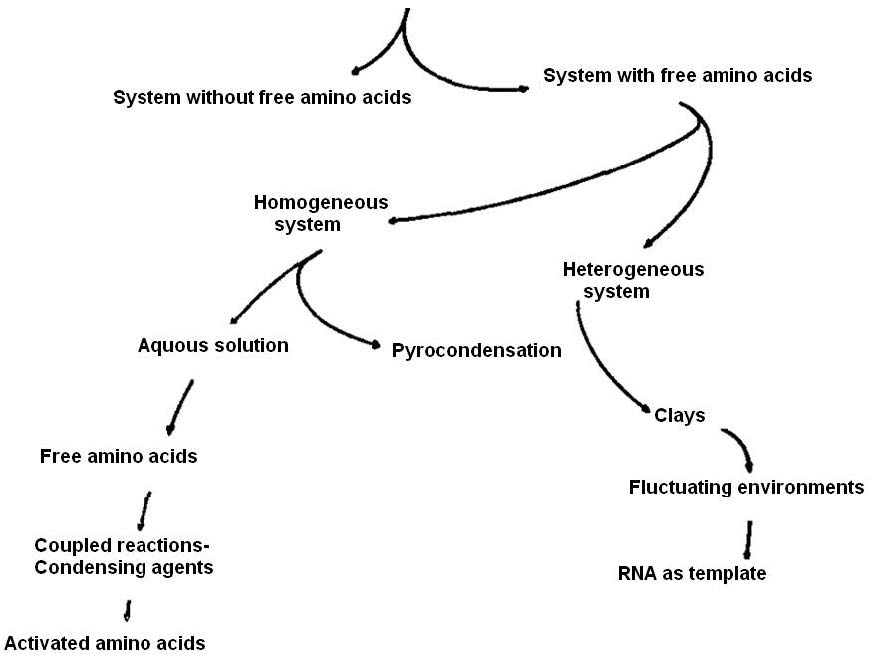Figure 1. Models for prebiotic synthesis of polypeptides.

2.2. Models in the absence of free amino acids

Due to the limitations of the synthesis of polypeptides using free amino acids, another approach to these syntheses was studied, starting with polymeric material that forms easily in prebiotic experiments. One example of this approach was made starting with the thermal polymers of HCN (Matthews et al., 1984).

Another approach is from the polymerization of alfa-aminonitriles (Fox and Dose, 1977).

3. Mathematical models in the prebiotic synthesis of polypeptides

Our subject study has been the oligomerization of amino acids under prebiotic conditions (i.e. under plausible conditions thought to have existed in the primitive Earth, before the emergence of life) using theoretical means.

In particular, we have been studying short sequences of oligopeptides yielded in the thermal polycondensation of a mixture of L- and D-α-amino acids, reported by experimental workers. A main premise of our approach is that the reactivity among monomers is different, as experimental results suggest. Namely, it has been reported that the thermal anhydrous synthesis of tri-peptides involving glutamic acid, glycine, and tyrosine produced only two tri-peptides. The formation of 36 tri-peptides is expected under an a priori assumption of an even probability of reaction between different amino acids (Fox et al., 1977; Nakashima et al., 1977). (We remark that these authors studied only tyrosine containing tri-peptides). Furthermore, a mechanistic study of this reaction has been performed (Hartmann et al., 1981).

We have looked into experimental systems claimed to produce biased polymers in composition, produced under plausible prebiotic conditions. We have examined thermal oligopeptides that have been studied extensively by Fox and collaborators (for an overview, see Fox and Dose, 1977). Fox proposed several decades ago that the reactivity between different amino acids is not even. He called this characteristic the principle of self ordering of amino acids.

We have other reasons to believe in a random but biased synthesis. In organic chemistry, in the presence of two monomers M1 and M2, and their respective free radicals, M1• and M2•, the propagation reaction is described as making use of four kinetic constants: k11 and k12 for reactions M1•+Mi, with i = 1, 2 and k21 and k22 for reactions M2• + Mi, with i = 1, 2. Of course, k11 ≠ k12 ≠ k21 ≠ k22(Katime, 1994). Such conditions would lead to the synthesis of biased and random polymers and not to purely random polymers. (Another manner to refer to biased and random oligopeptides is to call them oligopeptides with limited randomness).

In this work, we consider the polymerization of amino acids via a dehydration-condensation reaction. From the electric standpoint, all amino acids have identical amino and acid groups. They only differ in the electrical properties of the residue group. It is this group which determines the electrical properties of an amino acid. We adopted the Dickerson and Geis (1969) classification of amino acids — into polar positive (p+), polar negative (p), neutral (n), and non-polar (np) — which is an electrostatic or electromagnetic classification (the latter, when the charges are moving, which is usually the case). Such electromagnetic classification is important because we are focusing on possible chemical reactions between amino acids. In chemical kinetics, it is important to consider the electromagnetic nature of the reacting species. For example, we may have a reaction between an ion and a molecule (ion-molecule reactions which are very effective and fast), or a quite different reaction between two nonpolar molecules. It is with such ideas in mind that we adhere to this classification of amino acids.

4. Biased phenomena is a necessary condition for life’s origin

The relevance of biased oligomers to the emergence of life cannot be overemphasized. Consider a set of oligomers {n} and assume it embodies a pristine and close to minimum living chemical system (Mosqueira, 1988). Now take account of the following premises applying on {n}. (1) Plurality. Each single oligomer is assigned a single function to the global task; thus we need a set {n} of oligomers. (2) Simultaneity. It is assumed that the set {n} is located together at some physical space in order to be kinetically connected. In fact, the reconsideration of previous definitions of life underline spatially defined systems as an important feature for the emergence of life (Luisi, 1998; Ruiz-Mirazo et al., 2002). (3) Number of participating oligomers. It falls into the range 8n14. (4) Degree of polymerization x. It is assumed x around 40 (monomers). (5) Alphabet a. A two letter alphabet has been adopted, i.e., a = 2. It has been estimated (Mosqueira, 1988), using simple probability theory, that the supposition of an even probability of reaction among monomers would render {n} without any chance of reproduction. In other words, under the condition of equal probability of reaction among monomers, the number of possible sets {n} is so big that there is no chance to reproduce a given set {n} again. For this reason it is concluded that an unequal (or biased) probability of reaction among monomers is an indispensable condition for the reproduction of {n}.

5. A preview of Markov Chains

Before going into the formal presentation of our model in the next section, we give a simpler overview of it. We choose to use Markov Chains because we are facing a process immersed in chance events — i.e., the reactivity of amino acids among themselves — and because it fits well with this type of problem. A process or succession of events developing in time in which the result in any stage depends on chance is called a random or stochastic process. A classic and simple example of stochastic process is a succession of Bernoulli trials, in which there are exactly two chance events (results) that exclude mutually. For example, the two possible outcomes of flipping a coin, the amount of people in excess of a certain age and those that do not meet this condition, and so on. There are only two possible results that exclude each other. We notice that in Bernoulli trials, the result of the last event does not affect the result of the next chance event, that is, the result of flipping a coin does not affect the result of the next throw. In other words, we may say that this kind of stochastic process do not have any memory of previous events. However, for most stochastic process each result depends on what happened in the previous stages of the process. That is, such processes have some memory of previous events. For example, the weather of a certain day is not completely random, but it depends to a certain extent on the weather of previous days.

In the case of a stochastic process that depends on several previous results, the simplest case is the one that only depends on the result of the previous stage and not on anything else that had happened previously. To such stochastic process we call a Markov process or a Markov Chain and it is stated with matrix equations. It is a chain of random events happening in time, and each event is bound to only the previous one.

At each stage in the process, there is a finite number of events that can occur. These are the possible states of the system. Now, let us relate this with the classification of amino acids we will use. Our system has only four possible events: polar positive (p+), polar negative (p-), neutral (n), and non-polar (np). We will consider transitions probability from and to any of these four states. So, in total we have 16 possible transitions expressed in a matrix, which is called the transition matrix (see Equation 5). Remember that in a Markov Chain there is no concern of states of the system that had happened previously.

Another important remark: We are considering the transition probability as equivalent to the reactivity probability among such electric groups, taken pairwise (as in a typical bimolecular reaction). That is, an orthodox interpretation of a transition matrix in a Markov Chain is that a matrix element pij signifies the probability that an entity i becomes an entity j. In our approach, we interpret pij as the probability of chemical reaction between entities i and j.

Notice that every row in that matrix is depicting all possible transitions given a starting state of the system. For example, the first row of Equation 5 represents all possible transitions starting with a p+ species (given four possible events of the system): p+p+, p+p- , p+n, p+np. As there are no more possible transitions, the sum of the transition probabilities should sum unity (this condition is expressed in Equation 1). The same can be said with respect to the other rows of Equation 5.

Once a Markov process begins, there is a huge ramification of possible results. As an example, consider again the first row of the transition matrix 5 and represents two transitions:

In Figure 2 we start in the left with one possible event, that is p+, (we could have started with any of the other three events: p-, n, or np). If the variable k denotes the stage of the system (with k = 0, 1, ..., n), then this is the k = 0 stage. Later, it is considered the transition to any other of the four possible events in this system. This would be the k = 1 stage. To each transition it is associated with a transition probability (see Equation 1). As we said above, there is a total of 16 possible transition probabilities (see Equation 5). The higher values of the matrix elements correspond to interactions that are known to be more intense from physical chemistry (for example p+p- or p-p+), and lower values correspond to weaker interactions (for example nnp or p+p+). In a later section we will propose the numerical values for such transition probabilities.

We come back to Figure 2. Up to this moment we have passed through two stages. Let us interpret them from the chemical point of view. The first stage (k = 0) was the (arbitrary) initial event (the monomer p+). To arrive at a second stage (k = 1), it is necessary to use all four transition probabilities that are facing p+ towards the four possible events (p+, p-, n, np; these are the first row of matrix 5). From the chemical point of view, this is equivalent to considering the probability of the synthesis of a dimer (in fact, there should be four possible dimers synthesized at this stage, in different amounts). Afterwards, we arrive to the third stage (k = 2) (this is represented in the extreme right of Figure 2). It requires a second transition and it will use any of the 16 possible values of the transition probabilities from Equation 5. For stages k≥ 2, these 16 transition probabilities will be used again and again to calculate subsequent states of the system (see Equation 3).

We may say something in respect to the number of different oligomers produced and the number of monomers composing such oligomers. By inductive reasoning, we realize that the number of possible oligomers synthesized is given by 4k. That is, at stage k = 0, we have one initiator (= 40). At stage k = 1, we have presumably 4 (= 41) dimers synthesized. At stage k = 2, we have presumably 16 (= 42) trimers synthesized (Figure 2 illustrates up to this point). At stage k = 3, we have presumably 64 (= 43) tetramers synthesized, and so on. The number of monomers in the oligomer is given simply by k + 1 (except when k= 0).

As time elapses, the system arrives to a steady state, that is, a state in which the system does not change any more in time (see Equation 7). This is analogous to the steady state attained in a differential equation, it is the condition dy/dt= 0, when the variable y does not change in time anymore.

A friendly introduction to Markov Chains may be found in Arya and Lardner (1985).

We now proceed to make the formal presentation of our model.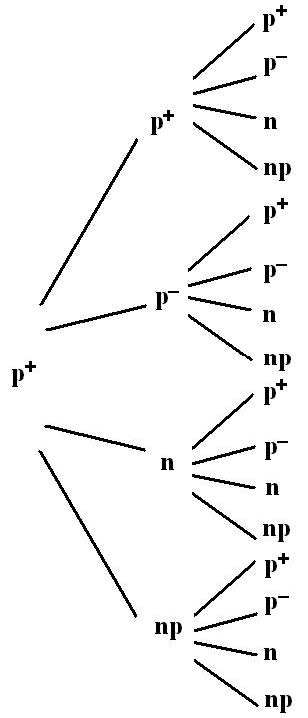Figure 2. An example of a given branching of a stochastic process in a Markov Chain.

6. The model

A finite Markov Chain is defined as follows (see for example Moran, 1984). Consider events that can occur at successive discrete stages and denote them by a variable, k, which can take the values 0, 1, ..., n. At each stage, a finite number of events E1, E2, ..., En can occur. These are the possible states of the system. At each stage k + 1, we suppose that the events E1, ..., En occur with certain probabilities, which depend only on the events that occurred at stage k and not on anything that had happened previously. We express pij for the probability of Ej to occur at stage k + 1 conditional on Ei having occurred at stage k.

The set of quantities, pij, i = 1, ..., n, j = 1, ..., nknown as the transition probabilities, are non-negative, and satisfy the conditions.(1)

The main assumption is that the transition probability of incorporating the n + 1 free amino acid into the oligomer is influenced only by the interaction between the incoming monomer and the reactive end of the oligomer, and is not influenced by any other previous monomers n - 1, n - 2, ... already bonded in the n-oligomer.

If the probabilities of the events E1, ..., En at any stage k are denoted by p1(k), ..., pn(k), for this state matrix after kstages, we have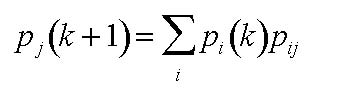(2)

and these equations can be written in the matrix form

 p(k +1) = p(k)P (3)

where p(k) is a row vector (or 1 x n matrix) whose elements are p1(k), ..., pn(k) and P = (pij) is an n x nmatrix and is known as the transition probability (or reactivity, or stochastic) matrix of the system.

Let us define a 1 x n initial state matrix (or an initial state row vector) p(0).

By applying Equation 3 repeatedly we see that

 p(k) = p(0)Pk (4)

where kis an integer.

Now, we assume different electromagnetic interactions between the reacting monomers (amino acids). To that end, in accordance with Dickerson and Geis (1969), there are four groups of amino acids: polar positive (p+), polar negative (p-), neutral (n), and non-polar (np). So, we arrange all possible electromagnetic interactions into a 4 x 4 Pmatrix.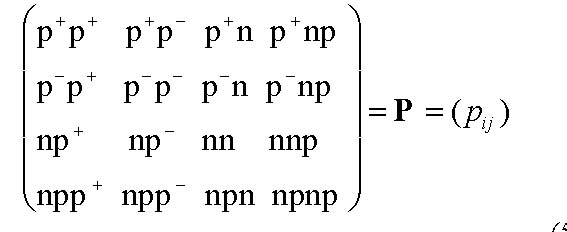(5)

Thus, for example, the element p13 is equal to p+n and it describes the interaction of a residue p+given that the last monomer in the oligopeptide is a residue of the class n. The rest the matrix elements in 5 are interpreted in a similar fashion.

Equation 5 may reduce its rank in case there are less than four groups of amino acids. That is, if there are only three groups of amino acids, then matrix 5 becomes a 3 x 3 matrix. Likewise, if there are only two groups of amino acids, it becomes a 2 x 2 matrix, and with only 1 group of amino acids, it becomes reduced to a 1 x 1 matrix. This is necessary in order to maintain in every instance a stochastic transition matrix (Equation 1).

The state of the system is represented at any stage kby a matrix of the state of the system that is a row matrix with four elements:

 (p+ p–n np) (6)

As time elapses, such initial state attains a steady state. Such state may be calculated by the following equation:

 p(k) = p(k)P (7)

This equation states that the row vector of a given stage is the same as the row vector of the following stage. This of course is the steady state condition in which the state of the system does not change anymore as time elapses. In our experience, this state seems to appear once k has attained a sufficiently large value (i.e., k is not greater than 6–11). This state persists to all subsequent stages, as long as the process is sustained, i.e., in our case, as long as the chemical process of polymerization is sustained. To calculate the steady state row vector, we should use Equation 7 plus the probabilistic condition expressed by Equation 1.

Finally, we should make a succinct comment on the interpretation that we give to pij in Equation 5, which slightly differs from an orthodox interpretation of a transition matrix in a Markov Chain. In a Markov Chain, a matrix element pijsignifies the probability that an entity i becomes an entity j. In our approach, we interpret it as the probability of chemical reaction between entities i and j, to become a dipeptide ij, and so on to form oligopeptides. Furthermore, the entries at each row of the transition matrix 5 represent unknown relative reactivities of one of the four types of amino acids considered with all the other types, including itself. This is the summary of the model up to this point.

6.1. Symmetry of the transition matrix 5

In respect to the symmetrical elements in Equation 5, (i.e., pij = pji), apparently, we should assign the same numerical value, as it might be thought that it is the same phenomenon if object P interacts with object Q, or if object Q interacts with object P. However, a careful examination of this situation leads us to the conclusion that in chemistry, the symmetrical case is the exception, and the asymmetrical situation is the rule. To illustrate this aspect, we will use specific members of amino acids to form a dimer. Then, let us use lysine (p+) and glycine (n). Then, we construct Gly-Lys and Lys-Gly dimers.

It can be seen from Figure 3 that neither object is symmetrical. These dimers possess a different charge distribution and therefore are not equivalent. Using basic chemistry and enzyme biochemistry, it can be shown that both dimers react differently in chemical and enzymatic reactions. Such condition suggests that the symmetrical elements in matrix 5 do not have an equal value. That is, we will assume they don't.Figure 3. Gly-Lys and Lys-Gly dimers.

6.2. Mathematical results with only two kinds of amino acids

We have applied the reactivity matrix 5 to particular reacting systems in which only two different species participate. Three distinct situations may arise depending on the specificity of the reactants (Mosqueira et al., 2002).

6.2.1. Diagonal interactions of the reactivity matrix are neglected

Matrix 5 becomes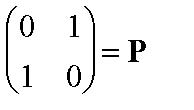In this case, if we assign a state matrix of the system at stage k = 0 or any other subsequent k as: (x y), where x and y are non-negative and satisfy the condition x + y = 1. We may verify then that at the k + 1 stage the state matrix is (y x); at k + 2 stage it is again (x y), and it continues in such alternate manner as long as the chemical process may proceed. So, in this case we encounter a sustained oscillatory steady state.

6.2.2. Equal symmetrical interactions. Diagonal interactions are non-null and small

In this situation we allow a pairwise interaction with similar, non-null elements. Supposedly, such elements are much smaller than the symmetrical interactions. As an example, we propose the following transition probability matrix: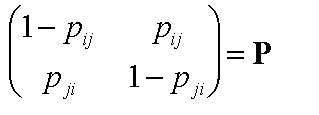And use some initial matrix in accordance, as for example (x y), where x and yare nonnegative and satisfy. Under such conditions we find a series of transient state matrices characterized by a damped oscillatory behavior that approaches the steady state matrix (0.5 0.5).

6.2.3. Symmetrical and diagonal interactions equal to 0.5

The steady state properties of a transition probability matrix symmetrical and diagonal interactions equal to 0.5, applied to an initial matrix, for example (x y), where x and y are non-negative and satisfy , are such that in a single stage (i.e., in k= 1) arrives to the steady state matrix (0.5 0.5).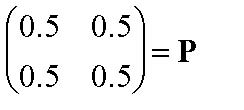In summary, with the exception of case 6.2.1, all steady states arrive to the steady state matrix with two matrix elements equal to 0.5. Such a peculiar situation arises from the symmetrical form of P that we have used as examples. Thus, in the framework of only two types of amino acid interactions, the constraint implies that we are giving the same probability to both types of amino acids to appear in the sequence. For this reason, either after a short transient (i.e., k = 1) or a longer one (k= 12), we arrive to a steady state matrix with two elements equal to 0.5.

7. Chemical aspects of the thermal prebiotic oligomerization of amino acids

To apply the present mathematical model, we have to know the reaction mechanism of the chemical transformation. In this manner, the electrical character of the reacting species at every stage can be assigned correctly. We will show in what follows the diketopiperazine reaction (Mosqueira et al., 2008), which is the mainstream reaction mechanism under thermally dehydrating conditions. In turn, we will recount the initiator and elongation stages of the diketopiperazine reaction.

7.1. The initiator stage

Several decades ago, it was experimentally established that to polymerize amino acids under anhydrous thermal conditions, there must be a sufficient proportion of at least one tri-functional amino acid, such as aspartic acid, glutamic acid, or lysine (Harada and Fox, 1965). Otherwise the mixture of amino acids does not polymerize and are spoiled by charring. When glutamic acid is used as the tri-functional amino acid, then the initiator is pyro-glutamic acid (pyrGlu), as it has been determined on the basis of chemical analysis (Fox et al., 1977; Melius and Hubbard, 1987).

7.1.1. Glutamic acid as initiator

Under the perspective of our stochastic and electric charge model, it is easy to explain the synthesis of pyrGlu from Glu. A glutamic acid molecule has three centers of charge (two negatives and one positive) with no predominance of either of them. We conceive the formation of pyrGlu as an internal cyclization process that proceeds readily, because in the same molecule we have the amino and carboxylic groups nearby (p+ and p-species, respectively) that by internal rotation react rapidly to get pyrGlu. The product of this intra-reaction has a concentrated negative charge on it (see Figure 4), giving rise to a powerful initiator for the oligomerization reaction.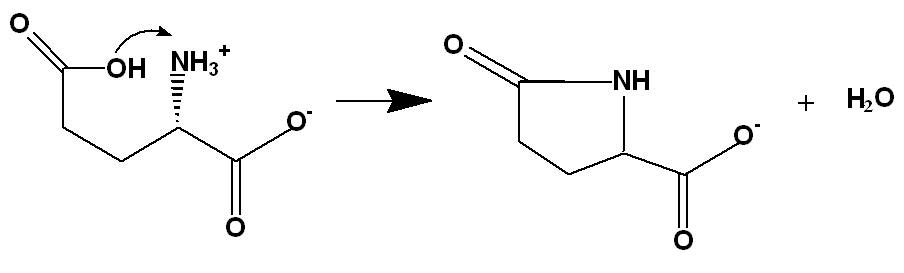Figure 4. Internal cyclization of glutamic acid (Glu) to produce pyroglutamic acid (pyrGlu).

7.1.2. Lysine as initiator

Harada (1959) studied the homo-polymerization of lysine, and some other co-polymerizations. He reported that the free DL-lysine converted to its liquid lactam at 150 – 170 ºC with vigorous evolution of water vapor (see Figure 5), and homo-polymerized at 180 – 230 ºC. There seems to be a two-stage reaction mechanism. In the first step there is an internal cyclo-dehydration of lysine (A), giving rise to a lactam with a net positive charge (B). That is, a tri-functional amino acid (A) is converted to a mono-functional amino acid (B). This is another instance of internal cyclization, analogous to the formation of pyrGlu from Glu. Of course, in this case the cyclic molecule produced has a concentrated positive charge on it, giving rise again to a powerful initiator for the oligomerization reaction, which in fact is able to polymerize itself (Harada, 1959).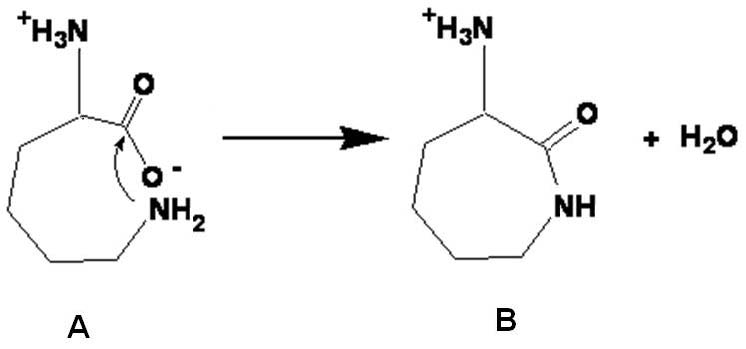Figure 5. Internal cyclization of lysine.

7.2. The elongation stage

Let us look in more detail at the synthesis of pen tamers by means of the diketopiperazine reaction. The reaction mechanism for the synthesis of trimers containing tyrosine is known (Hartmann et al., 1981). From such work, it is clear that a main route to oligomerization is through the chemical reaction of diketopiperazine molecules with other species. Molecules of diketopiperazine arise from the cyclodehydration of two amino acids to form a cyclic diamide (Figure 6), where R1 and R2 are the residue groups of the reacting amino acids. We call such reaction an external cyclization reaction (Mosqueira et al., 2008).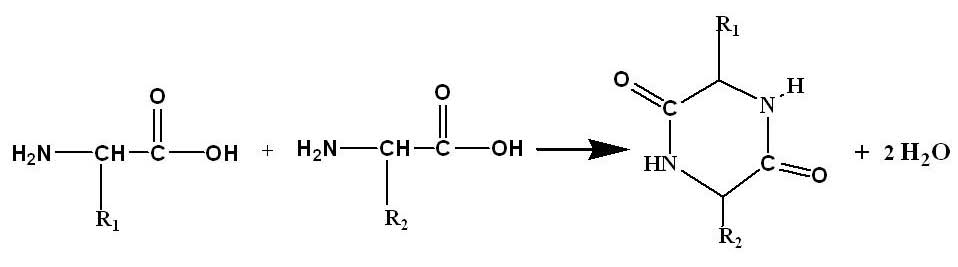Figure 6. Synthesis of a diketopiperazine molecule from the cyclodehydration of two amino acids with residue groups R1 and R2.

We now allow an initiator molecule, let's say pyrGlu (Figure 4), to react with a given diketopiperazine molecule with residue groups R1 and R2 (Figure 6) to yield two tripeptides (both tripeptides are p) (Figure 7)

We envisage that a diketopiperazine molecule with residue groups R1 and R2 is cleaved by pyroGlu and yields the two possible linear trimers pyroGlu–R1–R2 and pyroGlu–R2–R1 (Hartmann et al., 1981), as in Figure 7. Notice that both trimers reconstitute a free carboxylic group at their growing end (p-), equivalent to that of pyroGlu (p-). Then, both trimers may act as initiators (as pyroGlu) and react with another cyclic diketopiperazine to produce four pentamers, i.e., four pyroGlu–tetrapeptides. These pentamers may also react with another diketopiperazine, to continue as long as the reactants are present to produce only odd-mer oligopeptides (p). Besides, at the temperature of this reaction (180°C), we may expect that there is little stereoselective effect between R1 and R2 to obtain near equimolar amounts of both trimers. This conjecture has been proven to be correct with exact equimolar amounts when R1 and R2 are Gly and Tyr (Hartmann et al., 1981).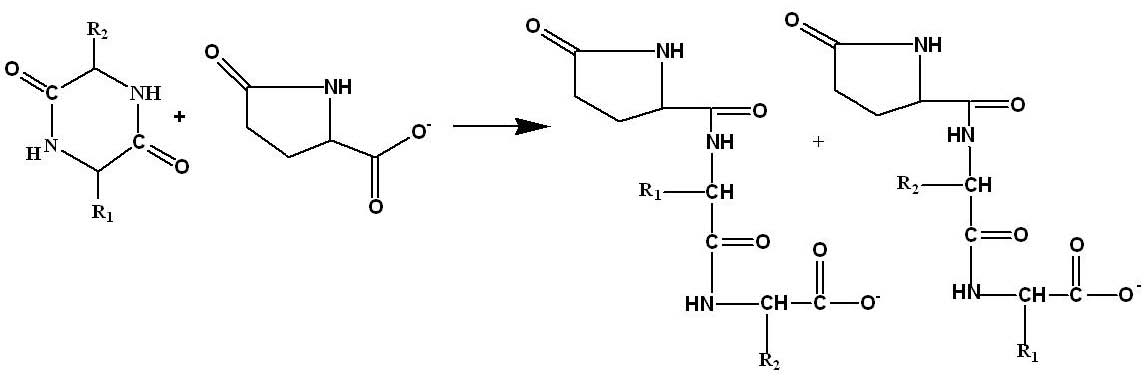Figure 7. Reaction of a diketopiperazine with pyroglutamic acid to synthesis two tripeptides with different sequence.

To analyze such a reaction mechanism from the perspective of our model, we should consider the electromagnetic nature of the reactive participating species at each stage. As we have said, the initiator (pyroglutamic acid) and the subsequent oligopeptides produced have a definite negative charge (p). However, the diketopiperazine molecules have two electromagnetic contributions: one arising from R1, the other from R2.

When residue 1 and residue 2 are, for example, non-polar, there is no doubt in considering this diketopiperazine molecule as a non-polar molecule as a whole. However, when residue 1 is polar positive (p+) and residue 2 is neutral (n), we reason that the more dominant electromagnetic interaction would be that arising from the polar positive group, and neglect the small contribution from the neutral residue. We consider then that this diketopiperazine molecule as a whole behaves as a polar positive species, as a first approximation. Another instance arises with not-so-obvious resolution. When residue 1 is neutral (n) and residue 2 is non-polar (np), we choose the neutral residue as the more prevalent electromagnetic influence, as it is more susceptible to being polarized than a non-polar residue (Feynman, 1964). Polarizability is an important characteristic to our objectives, as it may signify that a residue or a chemical species is more prone to participate in a chemical reaction. With such criteria in mind, in Table 1 we summarize the simplifications we performed from a combination of R1 and R2 attached to the diketopiperazine kernel (depicted as R1-diket-R2) to become a single electromagnetic residue group R0.

Table 1. A proposed electromagnetic simplification of a diketopiperazine molecule with two residue groups (R1 and R2) into a single dominant residue R0.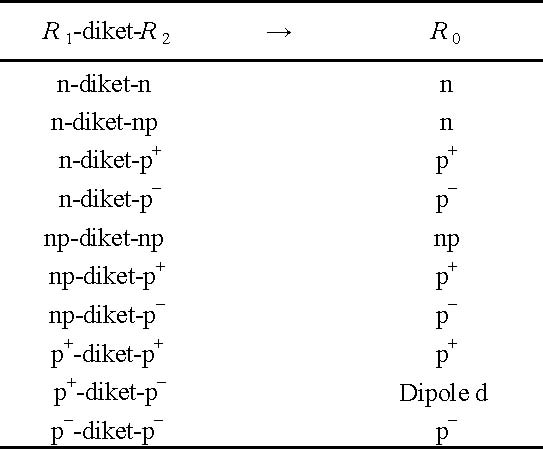This assumption will allow us to consider all possible pairwise interactions arising from the four-class classification of amino acids we have adopted (Dickerson and Geis, 1969), reacting via the diketopiperazine reaction. Clearly, our approach may be extended to include more (or less) than four classes of amino acids m by just expanding (or decreasing) the stochastic matrix 5 to an m x mmatrix.

A new situation arises when considering a diketopiperazine molecule formed with R1 = p+ and R2 = p(see Table 1). In this case, it is not possible to neglect one residue in favor of the other and a permanent dipole appears, which is different from any of the previous classes of amino acids. Nevertheless, from the perspective of the electromagnetic theory, such dipole may be dealt with as a single electromagnetic object (Feynman, 1964) and it is still possible to deal with such a case within the framework of our simple model. To that end, we should now deal with a 5 x 5 matrix as the new stochastic matrix 5 to include the dipole (d) interaction: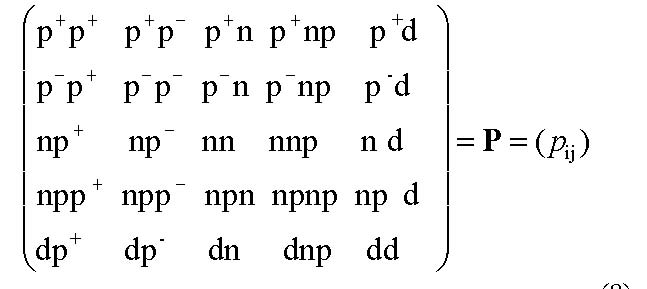(8)

Actually, all the elements of matrix 5 representing the interaction of a neutral (n) or non-polar (np) residue with a charged species, either p+ or p residues (p13, p14, p23, p24, and their corresponding symmetric elements), will give rise to a momentary interaction of an induced dipole. In the extended matrix 8, we might encounter interactions such as (permanent dipole)–(induced dipole), e.g., the element p53. A study in detail of such interactions – which we are not intending to perform – should take into account such phenomena.

8. Mathematical predictions of the model

There are several factors that contribute to reducing the variety of oligopeptides in the sequence space. They are the following: (1) the unequal probability of reaction among amino acids, (2) the existence of a Markov Chain steady state, (3) an observed independence of the initial conditions of the system, (4) the existence of a limited number of initiators for the oligomerization, and (5) production of only odd-mer peptides. Let us review these factors.

8.1. Unequal probability of reaction among amino acids

This condition appears to be self-evident. The probability cannot be the same to cause a reaction among pair p+ and p-than another pair of species like neutral (n) and non-polar (np). This factor decisively contributes to the synthesis of biased oligopeptides. The consequences of considering equal probabilities of reaction among amino acids highly contribute to make the emergence of life much less probable (Mosqueira, 1988).

8.2. The existence of a Markov Chain steady state

Equation 3 describes how the state of the system changes from the state k to the state k + 1. Similarly, as a differential equation attains its steady state when dy/dt = 0, a Markov Chain also attains a steady state (see Equation 7). This equation states that the row vector of a given stage is the same as the row vector of the following stage. So, the state of the system does not change any more as time elapses and this of course is the steady state condition. In our experience, this state seems to appear once k has attained a sufficiently large value (i.e., k around 5 < k < 12). This state persists to all subsequent stages, as long as the process is sustained, i.e., in our case, as long as the chemical process of polymerization is sustained.

The attainment of a steady state is an important mechanism that limits variability in oligomer sequencing. The state matrix is fixed in its steady state matrix and prevents it from roaming about over a huge sequence space that has been shown to exist (Mosqueira, 1988). In turn, the steady state itself appears to depend on two factors: The initial state matrix p(0) and the transition matrix P (see Equations 4 and 7). However, from our analysis, it appears that steady-state dependence is mostly on P. We have verified the independence of the initial conditions on the steady state from our previous work. Regardless of the initial conditions (concentration of participating amino acids), we arrive at the same steady state. In fact, this situation also occurs in differential equations. In summary, the independence of initial conditions in conjunction with the attainment of the steady state contributes to synthesizing biased oligopeptides.

8.3. The existence of a limited number of initiators for the oligomerization

The most common temperatures used to oligomerize α-amino acids under anhydrous conditions are 160 – 200 ºC, for approximately 9 – 12 h. It has been established that tri-functional amino acids (such as glutamic acid, aspartic acid or lysine) must be present in order to oligomerize ordinary amino acids with two functional groups (bi-functional amino acids); otherwise, the heating of purely bi-functional amino acids is recognized as a destructive treatment in which no oligomerization occurs (for a review see Fox and Dose, 1977).

Let us go again to the experimental result related to the synthesis of tyrosine containing trimers (Fox et al., 1977; Nakashima et al., 1977). This example will help to get an insight into the importance of an initiator to restrict variability of oligomers. The initiator is a derivative of glutamic acid: Pyroglutamic acid (pyrGlu). There is a kinetic basis to support that Glu is consumed rapidly to become pyroGlu, compared with other reactive species in the reaction (Hartmann et al., 1981). Then, trimer variability is reduced from 36 to only 6 trimers because pyroGlu should be the initiator of all possible trimers synthesized. There is a further reduction of three possible trimers because Glu cannot be in an internal position because it becomes pyroGlu quite rapidly (Mosqueira et al., 2000). This experiment illustrates the role of an initiator to get preferably biased oligomers.

8.4. The production of only odd-mer peptides

In Section 5 we outlined the reaction mechanism via the diketopiperazine molecule, which is a cyclic dehydrate condensation of two bi-functional amino acids. This mechanism allows only the production of odd-mer oligopeptides, with the exclusion of all even-mer oligopeptides. This fact surely reinforces the production of biased oligopeptides.

9. The mathematical predictions of the model with respect to heteropolymerization and homopolymerization

It is instructive to give a quantitative figure (thought approximate) for the interactions between different pairs of amino acids, for example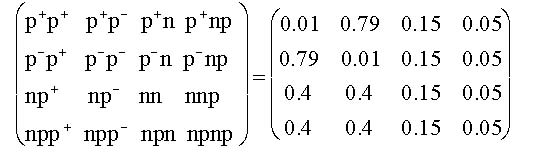(9)

Notice that the sum of values of the elements in each row is unity, according to Equation 1. Besides, the higher values of matrix elements correspond to interactions that we know to be more intense from physical chemistry, including p+p- or p-p+. On the other hand, lower values are given for interactions that are known to be much weaker, including nnp and p-p-.

From this perspective, it can be envisaged that contiguous alike charges or monomers will not be favored in a polymerization process under the conditions assumed in this work. On the contrary, it would be easier to unite contiguous charges of different polarity. With this background, we predict that for oligopeptides so produced, the heteropeptides would be more prevalent than the homoligopeptides (Mosqueira et al., 2012). Such conditions would be useful in the prebiotic environment because heteroligopeptides likely would have more pre-catalytic activities than homoligopeptides. We see, then, a natural emergence and predominance of complex polypeptides (co-polypeptides and hetero-polypeptides) over simpler homo-polypeptides. This is undoubtedly a valuable result.

10. Conclusions

In this work we have built a simple probabilistic model that limits the variability in sequences in a population of polymers (or n-mers) of amino acids. We propose that the polypeptides that were produced in a prebiotic environment were random, of course, but were biased and had a limited randomness, due to differences in the polarity of the participating amino acids, described in matrix 5. Our model has been able to justify some experimental results in respect to the synthesis of particular tripeptides. Thus, it may be applied further to test some stages of chemical evolution, as it was presented in the introduction of this work.

A population of biased oligopeptides makes the replication of a minimal chemical machinery compatible with life more accessible. However, a quantitative evaluation of the extent of bias induced has not been done so far. The extent and effectiveness of these constraints to reduce variability in sequences of oligomers remains an open question, because drawing conclusions from scarce experimental results and from very short oligomers (i.e. the tripeptides reported in Fox et al., 1977) is obviously insufficient.

Finally, of particular relevance to us is the prediction related to the nature of primordial oligopeptides. In the prebiotic world, in anhydrous environments with a steady source of heat, it would be more likely to have heteroligopeptides than homoligopeptides. This idea is unexpected as it might be thought that primitive oligopeptides were highly monotonous, with monomers being repeated throughout the sequence with little variation. This model instead suggests a primitive world with not so much monotonous sequences of oligopeptides, and with an implied catalytic potential.

Acknowledgements

This work was supported by PAPIIT grant IN 10513-RR10513 and CONACYT grant No. 168579/11.

References

Arya, J.C., Lardner, R.W., 1985, Mathematical Analysis for Business and Economics, 2ndRevised edition, Prentice-Hall Inc, 764 p.

Dickerson, R.E., Geis, I., 1969, The Structure and Action of Proteins: New York, Harper & Row Publishers, 120 p.

Feynman, R., 1964, Lectures on Physics: Massachusetts, Palo Alto, London, Addison-Wesley, 824 p.

Fox, S.W., Dose, K., 1977, Molecular Evolution and the Origin of Life: New York, Marcel Dekker, 370 p.

Fox, S.W., Melius, P., Nakashima, T., 1977, N-Terminal Pyroglutamyl Residues in Proteins and Thermal Peptides, in Matsubara, H., Yamanaka, T., (eds.), Proceedings of the Symposium on Evolution of Protein Molecules: Japan Scientific Societies Press, 111–120.

Harada, K., 1959, Thermal Homopolymerization of Lysine and Copolymerization with Neutral and Acidic Amino Acids: Bulletin of the Chemical Society of Japan, 32, 1007–1008.

Harada, K., Fox, S.W., 1965, Characterization of thermal polymers of neutral α-amino acids with dicarboxylic amino acids or lysine: Archives of Biochemistry and Biophysics, 109, 49–56.

Hartmann, J., Brand, M.C., Dose, K., 1981, Formation of Specific Amino Acid Sequences during Thermal Polymerization of Amino Acids: BioSystems, 13, 141–147.

Katime, I.A., 1994, Química Física Macromolecular: Bilbao, Spain, Servicio Editorial, Universidad del País Vasco, 407 p.

Luisi, P.L., 1998, About Various Definitions of Life: Origins of Life and Evolution of the Biosphere, 28, 613‒622.

Matthews, C.N., Lidicky, R., Schaefer, J., Stejskal, E.O., McKay, R.A., 1984, Heteropolypeptides from Hydrogen Cyanide and Water? Solid state 15N NMR Investigations: Origins of Life and Evolution of the Biosphere, 14, 243‒250.

Melius, P., Hubbard, W., 1987, Pyroglutamyl N-termini of Thermal Polyamino Acids: BioSystems, 20, 213–217.

Moran, P.A.P., 1984, An Introduction to Probability Theory: Oxford, Clarendon Press, 542 p.

Mosqueira, F.G., 1988, On the Origin of Life Event: Origins of Life and Evolution of the Biosphere, 18, 143–156.

Mosqueira, F.G., Negron-Mendoza, A., Ramos-Bernal, S., Polanco, C., 2012, Biased versus unbiased randomness in homo-polymers and copolymers of amino acids in the prebiotic World: Acta Biochimica Polonica, 59, 543–547.

Mosqueira, F.G., Ramos-Bernal, S., Negrón-Mendoza, A., 2000, A simple model of the thermal prebiotic oligomerization of amino acids: Biosystems, 57, 67–73.

Mosqueira, F.G., Ramos-Bernal, S., Negron-Mendoza, A., 2002, Biased polymers in the origin of life: Biosystems, 65, 99–103.

Mosqueira, F.G., Ramos-Bernal, S., Negron-Mendoza, A., 2008, Prebiotic thermal polymerization of crystals of amino acids via the diketopiperazine reaction: BioSystems, 91, 195–200.

Nakashima, T., Jungck, J.R., Fox, S.W., Lederer, E., Das, B.C., 1977, A Test for Randomness in Peptides Isolated from a Thermal Polyamino Acid: International Journal of Quantum Chemistry: Quantum Biolgy Symposium, 4, 65–72.

Negrón, A., Ramos, S., 2000, Chemical Evolution on the Early Earth, in Chela-Flores, J., Lemarchand, G., Oró, J. (eds.), Astrobiology: Origins from the Big Bang to Civilization: Kluwer Academic Publisher, 71‒84.

Oparin, A.I., 1938, The Origin of Life: New York, Macmillan, 270 p.

Ruiz-Mirazo, P., Peretó, J.G., Moreno, A., 2002, Proposal for a Universal Definition of Life (abstract). The 10thInternational Society for the Study of Origin of Life and 13th International Conference on the Origin of Life, 67.